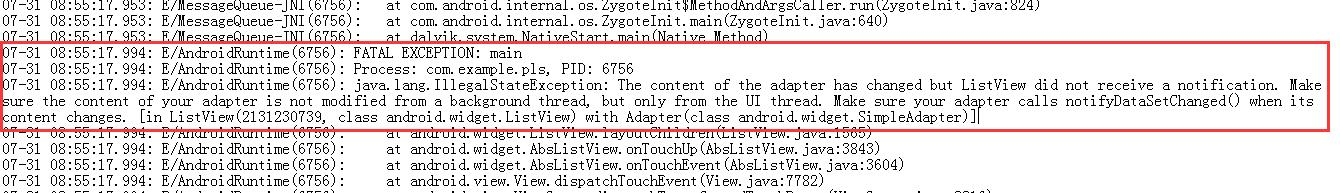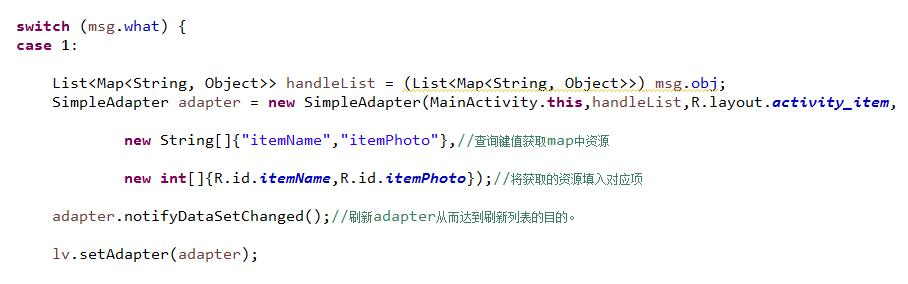ListView点击事件异常 10C`````` ListView lv = (ListView)findViewById(R.id.listView1);
lv.setOnItemClickListener(new OnItemClickListener() {
public void onItemClick(AdapterView<?> arg0, View arg1, int arg2,long arg3) {

TextView tv0 = (TextView)findViewById(R.id.textview0);
TextView tv1 = (TextView)findViewById(R.id.textview1);
TextView tv2 = (TextView)findViewById(R.id.textview2);
TextView tv3 = (TextView)findViewById(R.id.textview3);
ImageView img = (ImageView)findViewById(R.id.imageView1);

List<Map<String, Object>> listenList = tempList;
String name = listenList.get(arg2).get("itemName").toString();//从选中的item(map)中取出姓名
tv1.setText(" 姓名："+name);

int k = 0;
Sheet sheet = null;
try{
Workbook wb = Workbook.getWorkbook(xlsFile);
sheet = wb.getSheet(0);
/*Cell[] col1Arr = sheet.getColumn(1);

for(k=1;k<sheet.getRows();k++){
if(col1Arr[k].getContents() == name){
break;
}
}*/
for(k=1;k<sheet.getRows();k++){
if(sheet.getCell(1, k).getContents().equals(name)){//.equals(),面向对象方法
break;
}
}

}catch(IOException e){
e.printStackTrace();
}catch(BiffException e){
e.printStackTrace();
}

String cardID = sheet.getCell(0, k).getContents();//0列k行
tv0.setText(" 卡号："+cardID);

String age = sheet.getCell(2, k).getContents();//2列k行
tv2.setText(" 年龄："+age);

String depart = sheet.getCell(3, k).getContents();//3列k行
tv3.setText(" 部门："+depart);

String photoPath0 = Environment.getExternalStorageDirectory().toString() + "/picSrc/";
String photoPath = String.format("%s%s.bmp",photoPath0,name);
Bitmap bitmap = getLoacalBitmap(photoPath);
img.setImageBitmap(bitmap);

}
});
``````

4个回答